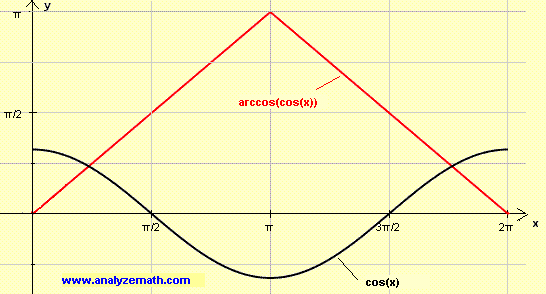# Find Derivative of f(x) = arccos(cos(x)) and graph it

A calculus tutorial on how to find the first derivative of f(x) = arccos(cos(x)) and graph f and f' for x in R.

## Graphs of cos(x) and arccos(cos(x))

Since the domain of f is R and cos(x) is periodic, then f(x) = arccos(cos(x)) is also a periodic function.
As x increases from 0 to π, cos(x) decreases from 1 to -1 and arccos(cos(x)) increases from 0 to π. In fact for x in [0 , π] arccos(cos(x)) = x. As x increases from [π , 2π], cos(x) increases from -1 to 1 and arccos(cos(x)) decreases from π to 0.
Since cos(x) has a period of 2π, arccos(cos(x)) also has a period of 2π. The graph below shows the graphs of arccos(cos(x)) and sin(x) from 0 to 2π.The graph below shows the graphs of arccos(cos(x)) and cos(x) over 3 periods.Domain of f: (-∞ , + ∞)
Range of f: [0 , π]

## Derivative of f(x) = arccos(cos(x)) and its Graph

f(x) is a composite function and the derivative is computed using the chain rule as follows: Let u = cos(x)
Hence f(x) = arccos(u(x))
Apply the chain rule of differentiation
f'(x) = du/dx d(arccos(u))/du = -sin(x) * (- 1 / √(1 - u
2))
= sin(x) * 1 / (1 - cos
2(x))
= sin(x) / √(sin
2(x))
= sin(x) / | sin(x) |
Below is shown arccos(cos(x)) in red and its derivative in blue. Note that the derivative is undefined for values of x for which sin(x) = 0, which means at x = k*π, where k is an integer. For these same values of x, arccos(cos(x)) has either a maximum value equal to π or a minimum value equal to 0.
Note that although arccos(cos(x)) is continuous for all values of x, its derivative is undefined at x = k*π.